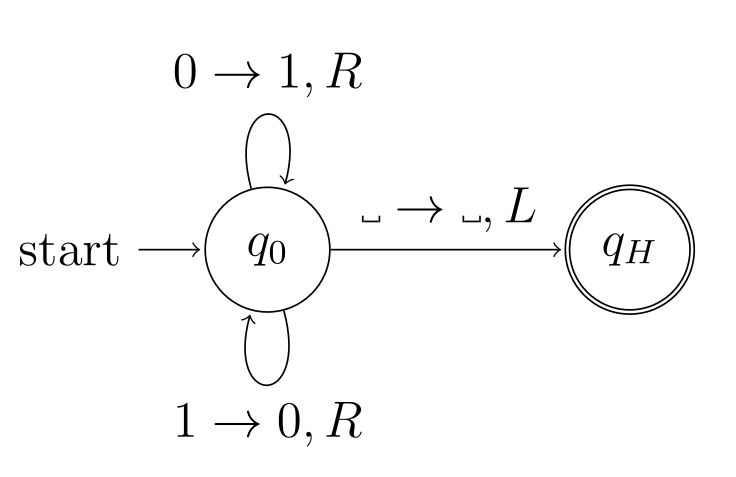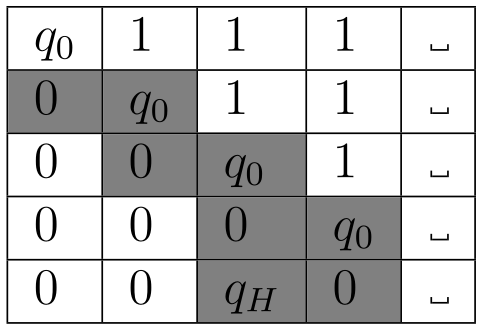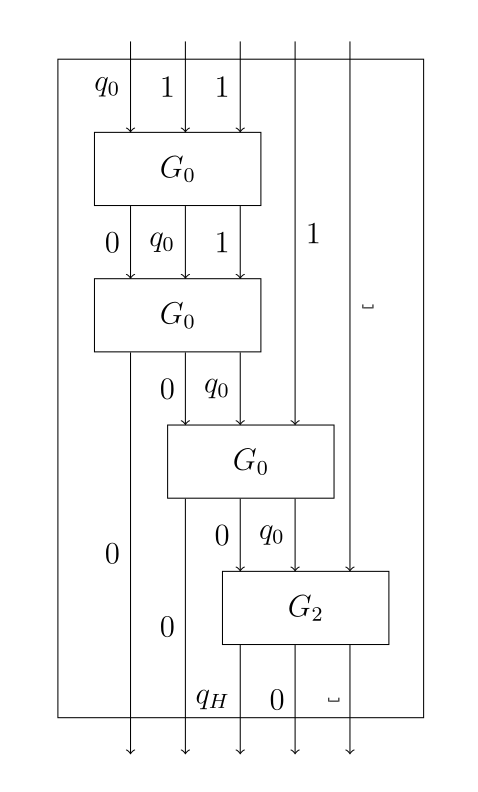Lets explore the necessity and use of Circuits as a computational model, and their use in MPC.

When I was first learning about garbled circuits, I had all sorts of primitive questions. I understood the “whats” but not the “whys”. This blog post is an attempt to introduce just enough complexity theory to justify the use of circuits in MPC protocols, in comparison to a hypothetical something else.

### Circuits are Turing Complete

This is either very suprising to you, or not at all. Looking at a circuit, it looks kind, not very smart. A glorified pachinko machine. But all real computers are built upon electric circuits. Circuits are Turing Complete, especially in the way that we care about. Recall the Cook-Levin theorem, the proof, not necessarily the result. For any Turing machine $M$ on input $w$, we will construct an equivalent circuit.

List the computation history in a tableu. At each row lies a sequential configuration of the machine. Create a circuit basis, a list of gates, for each transition of $M$. For each row of the tape, insert one gate appropriately. If you compose these, you get a circuit with depth the run-time of the machine, and width the space of the machine.

Lets demonstrate by example. Consider the following Turing machine:it will flip each bit on the tape and then halt. If we were to run the machine on input $111$, it would produce the following computation history

Notice that if we align these configurations into a tableu, that computation is local. At most two symbols change per level. Highlighted are the symbols which changed for that row, compared to the previous row.We can create a circuit basis from our Turing machine, with one gate per transition of the transition function. Here we only have three transitions. The gates of our basis will be three fan-in, three fan-out. Our circuit would look something like this:We have shown that for any halting computation, there exists an equivalent circuit. Therefore, circuits can do anything that Turing machines can. Sure, there is no circuit for an infinitely looping machine, but who cares about that. Even in complexity, the interesting problems are within subsets of the decidable languages; computations which halt.

Circuits are what are called, a non-uniform model of computation. A Turing machine takes on input of any size, but a circuit is part of an infinite circuit family, ${C_1, C_2, C_3, ...}$ where each circuit corresponds to accepting a different input size.

### Circuits are efficient

Now lets talk about practicality. We don’t use custom gates for creating circuits. You should be convinced that any such basis can be converted to a boolean circuit basis with only a constant overhead to the actual circuit. The DeMorgan basis is AND, OR, NOT, but in MPC we use AND, XOR, NOT, usually.

From the theoretical definition, you might assume that we would have to deal with a lot of circuits, one for each input size. As a theoretical definition, sure. But in practice no. The circuits are constructed of smaller subcircuits for things like, bounded 32 bit addition. You don’t need a new circuit for all possible input sizes because a 32 bit adder will safely, and correctly add inputs smaller than 32 bits. Even if you did need more than one circuit, your functionality could branch on the input length to two different subcircuits, meaning you would just have one larger circuit.

We measure circuit complexity as the asymptotics of the number of gates of $C_n$ as a function of $n$. Lets measure the circuit size from our previous proof. For a Turing machine $M$ of size $P$, which takes time $T(n)$ and space $S(n)$, you can trivially produce a circuit of size $P \cdot T(n) \cdot S(n)$. So any polynomial computation has a polynomial sized circuit. This can be improved to $P \cdot T(n)\log T(n)$. This is nontrivial to show, but perhaps believable, as most of the gates at each depth are identity ones.

The languages decided by polynomial sized circuit families are denoted by $\mathsf{P/poly}$. We showed that $\mathsf{P} \subset \mathsf{P/poly}$. We require the function $i \rightarrow C_i$ to be computable, otherwise there exist languages in $\mathsf{P/poly} \setminus \mathsf{P}$ such as $\begin{equation}\{1^{\langle M,w \rangle} \| M \text{ halts on } w\}\end{equation}$. Every unary language has a polynomial sized circuit family, and P only contains decidable languages. Requiring the map to be computable gives us equality. This should tell you that not only are circuits Turing complete, but they are efficient.

Circuits may seem large, take a look at some of the netlists. These are circuits for small useful programs, sorted topologically. Even though they seem large, how many invisible steps does you computer do for seemly atomic operations?

### I don’t know how to program a circuit

No one actually manually constructs boolean circuits, gate by gate. That would be boring. Instead code is written in a high level C like language and then compiled down into a netlist. The high level C like language is actually just overloaded operators and custom types. Ints from Bits and so on. You can even do bounded recursion. Its not done as the proof we described earlier, that was just to show the effiency and possibility.

Circuits have to be atleast as big as their input. The canonical example of the benefit of uniform models of computation is something like Google. Google takes as input the entire internet, so if they were a circuit, they would have to be atleast as big. Fortunately, we do not have to compile and store the entire circuit. Instead, they can be compiled and garbled on the fly. This is an engineering technique called streaming, and it enables computation of huge circuits, without having to have the entire circuit at once.

### Circuits are necessary

I will provide two persuasive arguments for the necessity of circuits.

Consider a hypothetical MPC system on any other kind of computational model. Consider the functionality:

Alice input: bit x
Bob   input: bit y

if x:
return 0
else:
long_running_time_function(y)


Suppose you were to try to create some kind of protocol to execute this securely. Bob might be able to determine Alice’s input bit just by measuring the wall clock time. A circuit, trivially, runs in constant time in the size of the circuit. If you were to encode this functionality as a circuit, and use garbled circuits based MPC, all branches would be computed and then only the correct one returned. Circuit based MPC would not be vulnerable to this attack, just by default. The hypothetical MPC system on some other computational model might fail here. Otherwise, it might have to do some kind of padding, which would make it at least as bad as circuit based MPC. This is a contrived example, but it shows how other computational models have issues which may not be obvious, and how circuits don’t, just as a default.

As a second argument, consider the value of circuits in building real, physical computers. I have tried building a Universal Turing machine out of bike parts for some time now. A Turing machine is about as simple as it gets, but mechanically, its still incredibly involved. You need some kind of programmable mechanism to move the head (or the tape) left of right. A mechanism which moved always left, or always right is so much simpler, but this would not be Turing complete. Having a mechanism which can move both left and right conditionally is very difficult to imagine. Thats just one part, you need some sort of statefulness, a way to measure, and act on that statefulness. You need programmability, and so on. The closest I have seen is this guy, who built an incredibly complex 3 state machine out of wood! Contrast this with circuits. You don’t need any moving parts, you just need a way to do a 4 row truth table, and to do it in a composable way. You don’t need to manage any state, thats implicitly done on the wires. You don’t need any moving parts, the circuits are so conceptually simple, its almost seems too good to be true. I don’t even mean electrical circuits. People have built circuits out of dominoes , marbles , water, I could go on. The simplicity of circuits is used to build real electric computers. This same simplicity is why circuit based MPC is such an obvious solution. When Yao invented garbled circuits, step zero wasn’t even “represent the functionality as a circuit”, it was just assumed the functionality was a circuit. At the time, the only concern was if you could perform MPC on any functionality, not just ones for specific protocols.

### Circuits are not limiting

Just because a boolean circuit looks like a direct acyclic graph, doesn’t mean you have to optimize from this level either. Its true that a lot of optimizations take this low level approach. The biggest optimizations are FreeXOR, which does zero communication for linear gates (like XOR, and NOT). Half gates and Threehalf gates do 2 ciphertexts and 1.5 ciphertexts per gate, respectively (down from four). These optimizations are independent of the circuit structure, but that doesn’t mean we have to be oblivious to the topology. In a series of papers, Dave Heath showed how to send garbling information only proportional to the longest branch. Its application is conditional on the structure of the circuit. There are some safe, obvious assumptions made. If you have several branches, these are each separate sub circuits and attached via something like a mutex. The part I want to stress here is the functionality is not studied like a messy circuit, but rather at the program level! Circuits don’t have branches unless their programs do. We don’t have to think at the circuit level to perform optimizations and achievements.

I want to conclude by saying that circuits have not limited us, rather they have enabled so many bigger things. They do come with some caveats, but these are inseperable from their advantages. You could argue we don’t even study circuits. We study programs with circuit representations.

### Other things

Circuits have a lot more interesting applications and study in complexity theory, but I wanted to present just enough to justify their use in MPC. I didn’t talk about arithmetic circuits, or FHE, or ZK, but similar ideas apply to those.

I am not suggesting that MPC is impossible without circuits. There do exist things along this line, such as GFSA or ORAM, but none are as simple in their construction.

Circuits used in practice are not actually generated from Turing machines. However, the idea, where at each sequential depth of the circuit, corresponds to a sequential state update, is essential.

The construction we gave can be reformulated to show that the language of satisifiable circuits is NP-complete.

Testing that a circuit is minimal is an extremely hard problem, and an active area of research. From my experience, given a circuit and trying to minimize it manually is not going to work ever. The circuit compilers don’t give any guarantees about minimality, but trying to improve upon them is futile.